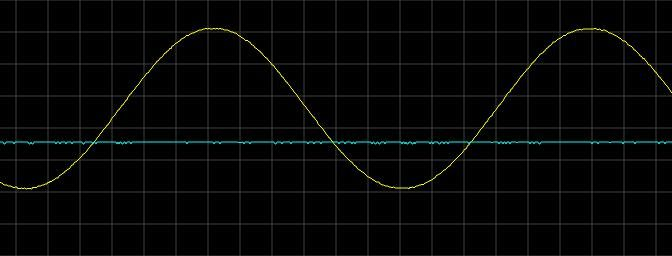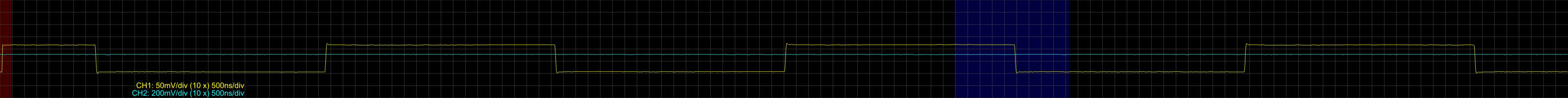# FL2000 as signal generator

First we need to generate some samples:

```import math
import struct

file = open("sin.bin","w")
i=0

while i<2*3.141:
i += 0.0001
val = math.sin(i)
#raw = int(127 - val * 127)
#raw = int(255 * val)
raw = struct.pack('b', val * 127)
file.write(raw)

file = open("square.bin","w")
lo = struct.pack('b', -128)
hi = struct.pack('b', 127)

for j in range (0, 100):
for i in range(0,1000):
file.write(hi)
for i in range(0,1000):
file.write(lo)

file = open("saw.bin","w")

for j in range (0, 100):
for i in range(-128,127):
val = struct.pack('b', i)
file.write(val)

```

Next is just “playing” and measuring them:

## Sinus

`./fl2k_file sin.bin`## Saw

`./fl2k_file saw.bin`## Square

`./fl2k_file square.bin`## 2 thoughts on “FL2000 as signal generator”

1.Ышктщ says:

This is a very good device

2.L29Ah says:

After reading your post I wrote a ncurses UI for using fl2k as a signal generator: https://github.com/l29ah/fl2k_signal_generator

This site uses Akismet to reduce spam. Learn how your comment data is processed.